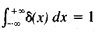Delta Function

Also found in: Wikipedia.

delta function

[′del·tə ‚fəŋk·shən]
(mathematics)
A distribution δ such that is ƒ(x). Also known as Dirac delta function; Dirac distribution; unit impulse.

Delta Function

(also δ function, Dirac delta function, or δ(x)), a symbol used in mathematical physics in solving problems in which there are point magnitudes (for example, point load and point charge). The delta function can be defined as the density of the distribution of masses, for which a unit mass is concentrated at the point x = 0, while the mass at all other points is equal to zero. Therefore, it is assumed that δ(x) = 0, when x ≠ 0 and δ(0) = ∞, while(“infinite splash” of a “unit intensity”). More precisely, the delta function is the name of the generalized function defined by the equalitywhich is valid for all continuous functions φ(x).

In the theory of generalized functions, the delta function is the name of the functional itself, which is defined by this equality.

References in periodicals archive ?
His topics include the delta function, the eigenfunction method, the adjoint operator, principal solutions, and Green's function method for the wave operator.
where [delta](x) is the Dirac delta function, and f is a given function such that [MATHEMATICAL EXPRESSION NOT REPRODUCIBLE IN ASCII] for some positive constant c.
5d(f), where we have used the even property of Dirac delta function.
Dirac delta function [delta](x - L) was introduced to describe a distribution of externally applied torque.
The basic idea is to spread out the delta function.
x] is the floor operator, [delta](x) is Dirac delta function and u(x) is the unit step function.
The frequency-domain transfer function of a system, and its output response to an impulse delta function located at t = 0, can be respectively written as
2](1/r) = 4[pi][delta](r), where [delta](r) = [delta](x)[delta](y)[delta](z) is the three-dimensional Dirac delta function.
x[less] than or equal to]z[less than or equal to]y] f (x, z) x y) and the identity is given by the delta function [delta](x, y) = [[delta].
It has been proved that the inverse operator is the solution of the related equation of the following form, in which the non-homogeneous term has been substituted by the Dirac's delta function :
Fisher, The delta function and the composition of distributions, Dem.
r]) is the 3-dimensional Dirac's delta function at the origin.

Site: Follow: Share:
Open / Close